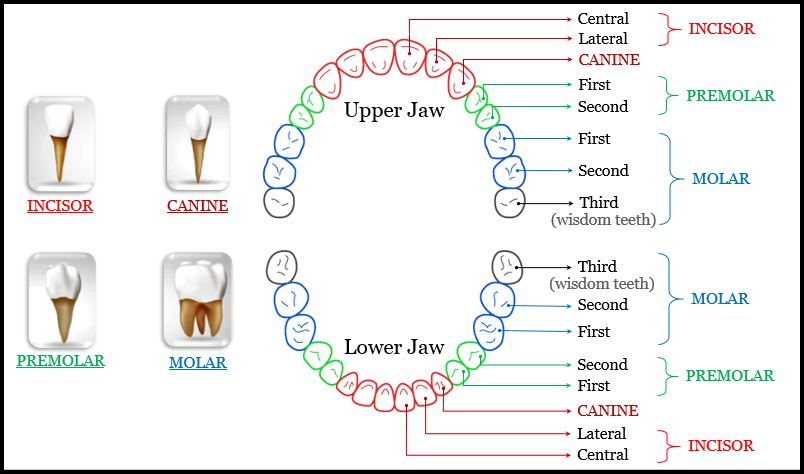# What is the dental formula, and what is the dental formula for children and adult?

The method of expressing the number and kind of teeth in mammals (such as man and animals) according to the arrangement is termed as the Dental Formula.

It is expressed using letters and figures, where the letters used in this formula are based on the 4 kinds of teeth which is represented as- i (incisor), c (canine), pm (premolar) or b (bicuspid), and m (molar), and the number of teeth in each jaw is written in the figure, with a fraction above the horizontal line showing the number of teeth, type of teeth in one side of the upper jaw, and those below the number of the same type of the teeth on one side of the lower jaw according to the arrangement.

The Dental Formula is expressed as:

$\displaystyle =\frac{( The\ number\ of\ each\ type\ of\ teeth\ in\ the\ half\ of\ the\ upper\ jaw)}{( The\ number\ of\ the\ same\ type\ of\ teeth\ in\ the\ half\ of\ the\ lower\ jaw)}$

Note:- In order to represent all of the teeth in the mouth, then multiply the whole formula by two, as the formula is for only one side of the jaw.

The dental formula for humans are categorized into two parts:-

1. The primary or temporary dentition: Present in children and termed as deciduous or milk teeth. It is composed a total of 20 teeth, 10 teeth on both the top and bottom jaw. Each jaw consists of 4 incisors, 2 canines, 4 molars, and 0 premolars.

The dental formula for deciduous or milk teeth is given as:

$\displaystyle i\ \frac{2}{2} \ c\ \frac{1}{1} \ p\ \frac{0}{0} \ m\ \frac{2}{2} \ =\ 10$  Where: i- Incisors, c- Canine, p- premolar and m- Molar

$\displaystyle \frac{2102}{2102} \ =\ 20$  (Total)

2. The permanent dentition: Present in adults. It is composed a total of 32 teeth, 16 teeth on both the top and bottom jaw. Each jaw consists of 4 incisors, 2 canines, 6 molars, and 4 premolars.

The dental formula for adult teeth is given as:

$\displaystyle i\ \frac{2}{2} \ c\ \frac{1}{1} \ p\ \frac{2}{2} \ m\ \frac{3}{3} \ =\ 16$   Where: i- Incisors, c- Canine, p- premolar and m- Molar

$\displaystyle \frac{2123}{2123} \ =\ 32$  (Total)